Next: Implementation Up: Mathematical background Previous: Improving the evaluation using   Contents

Single bisection mode

We may use the single bisection mode i.e. bisect only one variable at a time. Fives modes exist for determining the variable to be bisected, the choice being made by setting Single_Bisection to a value from 1 to 8

• 1 : we just split the variable having the largest width
• 2 : this mode is based on the smear function as defined by Kearfott : let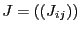be the Jacobian matrix of the system and let define for the variable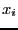the smear value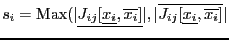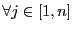whereis the total number of functions. The variable that will be bisected will be the one having the largest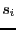. There is however a drawback f the smear function: let consider for example the equation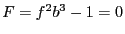where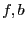are large identical intervals centered at 0. The derivative of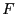with respect to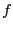is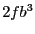and with respect to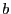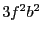: multiplied by the width of the interval we get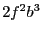and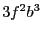. Hence the smear function forwill be in general larger than forandwill always be bisected until its width is lower than the desired accuracy. Another example in which the smear function is not the best choice is presented in section 2.4.3.4. However the smear function is very often the most efficient mode and should be privileged.
• 3 : this is similar to the smear function except that we take into account its drawback. To avoid bisecting over and over the same variable we impose that a variable may be considered for bisection only if the ratio of its width over the maximal width of the box is not lower than the variable ALIAS_Bound_Smear (default value 1.e-5).
• 4 : this mode is based on the Krawczyk operator: to determine which variable should be bisected we consider the box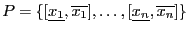. When dealing with the variablethe single bisection mode will lead to two new boxes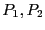. Let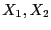be the middle point of these boxes We have seen (section 2.10) that a fundamental point of Moore test for determining the unicity of a solution in a box is that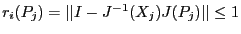. Thus we will consider in turn each of the variable and compute the value of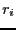for both. The bisected variable will be chosen as the one leading to the minimal value of all. However to avoid bisecting over and over the same variable we use another test: let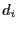be the width of the interval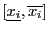and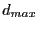be the maximum of all the. If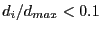we don't consider the variableas a possible bisection direction.
• 5: we use a round-robin mode i.e. each variable is bisected in turn (first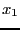, then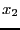and so on) unless the width of the range for the variable is less than the desired accuracy on the variable, in which case the bisected variable is the next one having a sufficient width
• 6: like mode 2 of SolveGeneral. ALIAS_RANDG may still be used to switch between mode 1 and mode 2 of SolveGeneral
• 7: like mode 2 of SolveGeneral except that it is assumed that the user has defined a simplification procedure that may allow to reduce the box directly within the bisection process
• 8: the variable are regrouped by groups of ALIAS_Tranche_Bisection elements. The bisection will look at each group in turn and bisect the first group that has elements whose diameter is larger than ALIAS_Size_Tranche_Bisection. When the element of the group have all elements whose diameter is lower than this threshold the bisection will consider the next group. If all elements of all groups have a diameter lower than the threshold the smear function will be used to determine which variable will be bisected.

The smear mode leads in general to better result than the other modes (but there are exception, see example in section 2.4.3.4). There is another mode called the mixed bisection: among thevariables we will bisect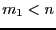variables, which will lead to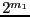new boxes. This mode is obtained by setting the global integer variable ALIAS_Mixed_Bisection to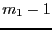. Here we will order the variables according to the value of their smear function (if the flag Single_Bisection is 2 or 3) or according to their width (for 1,4,5).Next: Implementation Up: Mathematical background Previous: Improving the evaluation using   Contents
Jean-Pierre Merlet 2012-12-20